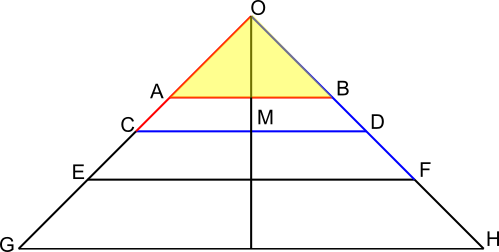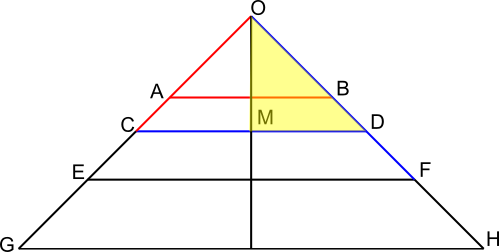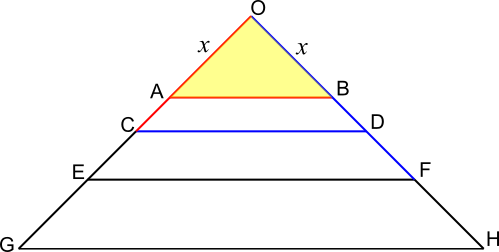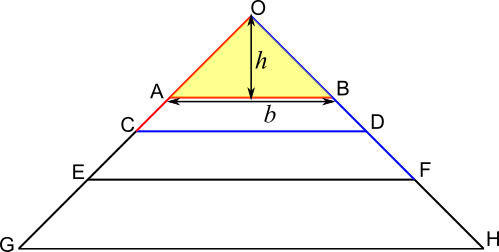#### You may also like### At a Glance

The area of a regular pentagon looks about twice as a big as the pentangle star drawn within it. Is it?### Six Discs

Six circular discs are packed in different-shaped boxes so that the discs touch their neighbours and the sides of the box. Can you put the boxes in order according to the areas of their bases?### Equilateral Areas

ABC and DEF are equilateral triangles of side 3 and 4 respectively. Construct an equilateral triangle whose area is the sum of the area of ABC and DEF.

# Towering Trapeziums

##### Age 14 to 16Challenge Level

T. Hakim from Annie Gale School in Canada defined a new point, $M$, as the midpoint of $CD$.

You had to understand the pattern of the triangles and the relationship between triangles $ABO$ and $ODM$.

This relation states that because the hypotenuse of $ODM$ is the same as $ABO$, and because it is an isosceles right triangle (so the other sides are the same),

$$ABO = ODM$$With this information, I was able to figure out the rest of the triangle, and even the $n^\text{th}$ trapezium with these steps!
1) last full smaller-looking triangle = half of the next triangle (in the first case, $OAB=\frac12OCD$)

2) multiply half-triangle by two to create full triangle (therefore $2\times$ size of the previous triangle) (in the first case, $OCD$ has area $2\times$ area of $OAB$ which is $2\times1=2$)
3) subtract larger from smaller to get trapezium (in the first case, $2-1=1$)

After solving 4 trapeziums, I noticed a pattern, which was doubling the last trapezium's size, which made it a lot easier to solve.

next trapezium = $2\times$ last trapeizum

Matt from Swindon Academy in the UK, Amrit from Hymers College in the UK and Pablo from King's School Alicante in Spain considered the sides $OA$ and $OB$ as the base and height of triangle OAB, since the angle between them is $90^\text o$.Amrit said:

Let $OA=OB=x$, then
$\frac12x^2=1$

Therefore $x^2=2\Rightarrow x=\sqrt2$

Let $AB=OC=y$, then by the Pythagorean theorem,

$2x^2=y^2$

Plugging in the value of $x$, we have $2\times2=y^2\Rightarrow y=2$

The area of triangle $OCD$ is $\frac12y^2=2$. Therefore the area of the trapezium is $2-1=1$.

Pablo and Matt continued using the same methods to find the areas of the next trapeziums and generalise. This is Pablo's work:
TRAPEZIUM 2
$OC = OD = 2$
$CD^2 = 2^2 + 2^2$
$CD^2 = 8$
$CD =OE= 2\sqrt2$

Area of $OEF = 2\sqrt2 \times2\sqrt2\times \frac12= 4$
Area of trapezium $CDFE =$Area$OEF -$Area$OCD = 4 - 2 = 2$ units$^2$

TRAPEZIUM 3
$OE = OF = 2\sqrt2$
$EF^2 = OE^2+ OF^2 = (2\sqrt2)^2+(2\sqrt2)^2$
$EF^2 = 8 + 8$
$EF = 4$
$OG = 4$

Area $OGH = 4\times4\times\frac12 = 8$ units$^2$
Area of trapezium $EFHG =$Area$OGH -$Area$OEF = 8 - 4 = 4$ units$^2$

NTH TRAPEZIUM
For the first trapezium, $ABDC$, we had to subtract the area of the triangle $OAB$ from triangle $OCD$. If we call $OA$ the 'first' length, $OC$ is the 'second' length.
$OA = \sqrt{2^1}$ [first]
$OC = 2 = \sqrt{2^2}$ [second]
this continues for $OE$ and $OG$

So the area of the trapezium was $\frac12 OC^2 - \frac12 OA^2$
If instead of $OA$ and $OC$ we had the $n^\text{th}$ and the $(n+1)^\text{th}$ lengths, we can
say that:
the area of the $n^\text{th}$ trapezium
$= \frac12\left(\sqrt{2^{n+1}}^2\right) - \frac12\left(\sqrt{2^n}^2\right)$
$= \frac12 \times 2^{n+1} - \frac12\times 2^n$
$= 2^n - 2^{n-1}$
$= 2^n\left(1 - 2^{-1}\right)$
$= 2^n\left(1 - \frac12\right)$
$= 2^n\left(\frac12\right)$
$= 2^n\left(2^{-1}\right)$
$= 2^{n-1}$

Amrit used similarity to find the area of the $n^\text{th}$ trapezium:
It is clear to see that all the trapeziums are similar.

Say $OA$ was length $y_0$ and $OC$ was length $y_1$, then $y_1=\sqrt2y_0$
If $CD=OE$ was $y_2$, then $y_2=\sqrt2y_1$

If we call $y_n$ the length of the $n^\text{th}$ parallel line, then $y_{n+1}=\sqrt2 y_n$

So $\dfrac{y_{n+1}}{y_n}=\sqrt2$

This is the scale factor between the sides of adjacent trapezia, so the area factor between them is $2$. We now need to find the area of the first trapezia to find a formula for the others.

The area of the first trapezium was $1$, so the area of the second trapezium is $2$, and the area of the third trapezium is $4$, and the area of te fourth trapezium is $8$, so
the area of the $n^\text{th}$ trapezium is $2^{n-1}$.

Guruvignesh and Adithya, also from Hymers College, considered the base and height of the triangle as horizontal and vertical.Firstly, we must use the formula for the area of a triangle: $\frac12b\times h=1\Rightarrow b\times h=2$

Then, they observed that splitting triangle OAB in half along the height $h$ creates two more right-angled triangles. The new triangles are also isosceles, so $\frac12b=h$.

So $b=AB=2$ and $h=1$ since the area is $1$ square unit.

Given that $AB=OC$, $OC= 2$ and similarly $OD=2$ since $OCD$ is a similar triangle to that of triangle $OGH$. Using the relative sizes of a 45 degree right angled triangle: $1:1: \sqrt2$ (this comes from $1^2+1^2=(\sqrt2)^2$),
$CD:OC=\sqrt2:1$
So $CD=2\sqrt2$

Because we now know that the height of each right angled isosceles triangle in this shape is half the base, the height of triangle $OCD$ is $2^\frac12$.
Thus, the height of the trapezium is $2^{\frac12} - 1$.
$$\begin{split}A&=\frac{a+b}2h\\ &=\frac{AB+CD}2\left(2^{\frac12} - 1\right)\\ &=\frac{2\sqrt2+2}2\left(2^{\frac12} - 1\right)\\ &=\left(\sqrt2+1\right)\left(\sqrt2-1\right)\\ &=1\end{split}$$ So the area is $1$ square unit.

For the next trapezium,
$OE = 2\sqrt2$
Using the relative sizes ratio for a right angled isosceles triangle:
$EF = 4$
Therefore the height of triangle $OEF$ is $2$
But the height of the trapezium is $2 - \sqrt2$
Area of trapezium $CDFE:$
$$\begin{split}A &= \frac12 (a + b) h\\ &= \frac12 (EF + CE) (2 - \sqrt2)\\ &= \frac12 (4 + 2\sqrt2) (2 - \sqrt2)\\ &=(2+\sqrt2)(2-\sqrt2) &= 2\end{split}$$

Adithya and Guruvignesh both created a table to show how the sequence continues:
 Term 1 2 3 4 5 Area 1 2 4 8 16

And they spotted that the $n^\text {th}$ term is equal to $2^{n-1}$.
The reason for this is because each trapezium is a similar shape to the one before. So, if we increase by scale factor $\sqrt2$ (due to the relative sizes of a right angled isosceles triangle), the area factor will increase by 2. Because $AF = SF^2$.